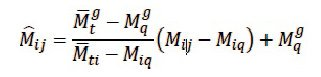# GATE normalization process explained

We all know Gate exam is of 100 marks. You must be worrying then how Gate is giving you a score on 1000 marks scale. In this article, we are clearing this doubt. This article will also help you in calculating your normalized marks on a scale of 1000 (if you have your gate marks ready).

## Gate normalization process

As we all know that there are 10 lacs + students who appear in 23 different engineering streams in Gate exam. Since the number of students is high in some streams, these engineering streams conduct exam in multiple shifts. The rest of streams have relative lesser number of candidates and therefore don’t require to be held in multiple sessions.  As there is a paper difference in each of these shift for same engineering stream, Gate board normalize marks of students keeping in check marks obtained by the student, marks obtained in that particular shift and marks obtained in all shift.

In Gate exam, the normalization process is the method used to convert the marks of students into normalized scores after computing according to the specifications prescribed by the GATE authorities. Normalization will be different for the single session and multiple session GATE papers. While the raw marks of the single session GATE papers will be used for computing the GATE scores, the actual marks for multiple sessions will be normalized and these normalized marks will be used to compute the GATE scores.

### Calculation of Gate normalized marks

Gate normalized marks are used for calculating ranks in various engineering streams, They are calculated on basis of performance of students in different shits. Here is an equation for calculating Gate normalized marks.

Equation 1Example:

Lets assume student marks = 60

Average marks of top 0.1% in ith session = 55

Average marks of top 0.1% in all sessions = 52

Sum of mean marks and standard deviation in ith session = 38

Sum of mean marks and standard deviation in all sessions = 34

Normalised marks = 18/17  x 26 + 34 = 61.53 marks

### Calculation of Gate score on the scale of 1000

In this equation,M = Marks obtained by the student in a single session, or normalized marks (from Equation 1) for multiple session

Mq = Qualifying marks for general category student

Mt = Mean marks of top 0.1% of students

Sq = 350

St = 900

Example

Now lets assume Mq = 25 , Mt =60 , M = 45

Marks on scale of 1000 = 350 + 550 x 20/35 = 664 marks

## How to calculate Gate rank

Gate ranks are always calculated on basis of normalized marks. Here is an example of rank and normalized marks and marks on a scale of 1000.Note that Ranks are calculated from normalized marks.

## Is there a Qualifying Cutoff marks in GATE and what is it?

To qualify Gate exam, a candidate needs to score above cut off marks. Gate guidelines suggest that minimum passing marks (cut off) in Gate exam for general category student in 25 marks or average marks + standard deviation (whichever is high). We have seen that due to the high value of average marks+Standard deviation, Gate board is using this value.

Here are cut off marks of some of the engineering streams in Gate exam 2017

 S.No. Paper Name Paper Code Qualifying Marks General OBC (NCL) SC/ ST/ PwD 1 Aerospace Engineering AE 34.4 30.9 22.9 2 Agricultural Engineering AG 25 22.5 16.6 3 Architecture and Planning AR 43.9 39.5 29.2 4 Biotechnology BT 27.9 25.1 18.5 5 Civil Engineering CE 26.9 24.2 17.9 6 Chemical Engineering CH 37.8 34 25.1 7 Computer Science and Information Technology CS 25 22.5 16.6 8 Chemistry CY 25.4 22.8 16.9 9 Electronics and Communication Engineering EC 25 22.5 16.6 10 Electrical Engineering EE 29.1 26.1 19.4 11 Ecology and Evolution EY 49.3 44.3 32.8 12 Geology and Geophysics GG 32.4 29.1 21.5 13 Instrumentation Engineering IN 37.1 33.3 24.7 14 Mathematics MA 29.4 26.4 19.5 15 Mechanical Engineering ME 34.7 31.2 23.1 16 Mining Engineering MN 45 40.5 30 17 Metallurgical Engineering MT 40.3 36.2 26.8 18 Petroleum Engineering PE 39.8 35.8 26.5 19 Physics PH 29 26.1 19.3 20 Production and Industrial Engineering PI 32 28.8 21.3 21 Textile Engineering and Fibre Science TF 32.2 28.9 21.4 22 Engineering Sciences XE 31.5 28.3 21 23 Life Sciences XL 29.9 26.9 19.9

## Conclusion

In the article, we have shared the process of normalization of Gate score and how the ranks are calculated. All the engineering colleges use Gate score (on a scale of 1000) for admission of students in different engineering streams.

If you have any problem in understanding Gate scores and marks, please reply to the comment box below. We will be happy to help you.

All the best.

Other important resources for Gate preparation

Syllabus and books for GATE

Gate paper pattern and cut off

Best IITs and NITs for doing MTech

GATE preparation calendar

Preparation strategy by GATE toppers

Preparation tips for Engineering Mathematics in Gate